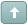Welcome Guest! To enable all features please Login or Register.Error

 Previous Topic Next Topic
 henry1224 #1 Posted : Saturday, April 16, 2005 3:20:00 PM(UTC)Rank: Advanced MemberGroups: Registered, Registered Users, SubscribersJoined: 10/29/2004(UTC)Posts: 1,394Location: Glastonbury, CTWas thanked: 1 time(s) in 1 post(s) This indicator is included in the Fibonacci trader program, I have adapted it to plot the weekly Support and Resistance lines on a daily chart. Plot them on the daily price chart, change the color of the top 2 lines to dark red and style to dots,the next two lines should be red and plot as dots the middle line is blue, the next 2 lines should be light green and plotted as dots, and finally the bottom 2 lines are dark green and plotted as dots A1:=BarsSince(DayOfWeek()=5)=1; A2:=BarsSince(DayOfWeek()=5)=6; CON:=If(BarsSince(A1)BarsSince(A2),1,0)); WH:=If(Con=-1,ValueWhen(1,DayOfWeek()=5,Ref(HHV(H,5),-1)),If(Con=1,ValueWhen(1,DayOfWeek()=4,Ref(HHV(H,4),-1)),ValueWhen(1,DayOfWeek()=5,Ref(HHV(H,5),-1)))); WL:=If(Con=-1,ValueWhen(1,DayOfWeek()=5,Ref(LLV(L,5),-1)),If(Con=1,ValueWhen(1,DayOfWeek()=4,Ref(LLV(L,4),-1)),ValueWhen(1,DayOfWeek()=5,Ref(LLV(L,5),-1)))); WCL:=If(Con=-1,ValueWhen(1,DayOfWeek()=5,Ref(C,-1)),If(Con=1,ValueWhen(1,DayOfWeek()=4,Ref(C,-1)),ValueWhen(1,DayOfWeek()=5,Ref(C,-1)))); BP:=(WH+WL+WCL)/3; D:=((WH-WL)/2)+BP; B:=BP-((WH-WL)/2); D1:=(WH-WL)+BP; B1:=BP-(WH-WL); SB1:=BP-((WH-WL)*.618); SB2:=BP-((WH-WL)*1.382); RB1:=((WH-WL)*.618)+BP; RB2:=((WH-WL)*1.382)+BP; RB2;D1;RB1;D;BP;B;SB1;B1;SB2;Wanna join the discussion?! Login to your Discussions forum accountor Register a new forum account.henry1224 #2 Posted : Saturday, April 16, 2005 3:21:26 PM(UTC)Rank: Advanced MemberGroups: Registered, Registered Users, SubscribersJoined: 10/29/2004(UTC)Posts: 1,394Location: Glastonbury, CTWas thanked: 1 time(s) in 1 post(s) This version just plots the last value of the Jackson Zones TF:=Input("1=hour 2=week 3=month 4=year ",1,4,3); NW:=If(TF=1,ROC(Minute(),1,\$)<0,If(TF=2,ROC(DayOfWeek(),1,\$)<0,If(TF=3,ROC(DayOfMonth(),1,\$)<0,ROC(Month(),1,\$)<0))); A1:=Cum(1); WH:=ValueWhen(1,Nw,Ref(HighestSince(1,Nw,H),-1)); WL:=ValueWhen(1,Nw,Ref(LowestSince(1,Nw,L),-1)); WCL:=ValueWhen(1,Nw,Ref(C,-1)); BP:=LastValue((WH+WL+WCL)/3); D:=LastValue(((WH-WL)/2)+BP); B:=LastValue(BP-((WH-WL)/2)); D1:=LastValue((WH-WL)+BP); B1:=LastValue(BP-(WH-WL)); SB1:=LastValue(BP-((WH-WL)*.618)); SB2:=LastValue(BP-((WH-WL)*1.382)); RB1:=LastValue(((WH-WL)*.618)+BP); RB2:=LastValue(((WH-WL)*1.382)+BP); A2:=LastValue(A1-BarsSince(NW>0)); If(A1=A2),RB2); If(A1=A2),D1); If(A1=A2),RB1); If(A1=A2),D); If(A1=A2),BP); If(A1=A2),B); If(A1=A2),SB1); If(A1=A2),B1); If(A1=A2),SB2);henry1224 #3 Posted : Saturday, April 16, 2005 3:22:15 PM(UTC)Rank: Advanced MemberGroups: Registered, Registered Users, SubscribersJoined: 10/29/2004(UTC)Posts: 1,394Location: Glastonbury, CTWas thanked: 1 time(s) in 1 post(s) This is the final revision for this indicator TF:=Input("1=hour 2=day 3=week 4=month 5=year ",1,5,4); NW:=If(TF=1,ROC(Minute(),1,\$)<0,If(TF=2,ROC(Hour(),1,\$)<0, If(TF=3,ROC(DayOfWeek(),1,\$)<0,If(TF=4,ROC(DayOfMonth(),1,\$)<0,ROC(Month(),1,\$)<0)))); A1:=Cum(1); A2:=LastValue(A1-BarsSince(NW>0)); WH:=ValueWhen(1,Nw,Ref(HighestSince(1,Nw,H),-1)); WL:=ValueWhen(1,Nw,Ref(LowestSince(1,Nw,L),-1)); WCL:=ValueWhen(1,Nw,Ref(C,-1)); BP:=LastValue((WH+WL+WCL)/3); RB2:=If(A1=A2),LastValue(((WH-WL)*1.382)+BP)); D1:=If(A1=A2),LastValue((WH-WL)+BP)); RB1:=If(A1=A2),LastValue(((WH-WL)*.618)+BP)); D:=If(A1=A2),LastValue(((WH-WL)/2)+BP)); MBP:=If(A1=A2),LastValue((WH+WL+WCL)/3)); B:=If(A1=A2),LastValue(BP-((WH-WL)/2))); SB1:=If(A1=A2),LastValue(BP-((WH-WL)*.618))); B1:=If(A1=A2),LastValue(BP-(WH-WL))); SB2:=If(A1=A2), LastValue(BP-((WH-WL)*1.382))); RB2;D1;RB1;D;MBP;B;SB1;B1;SB2;henry1224 #4 Posted : Saturday, April 16, 2005 3:23:08 PM(UTC)Rank: Advanced MemberGroups: Registered, Registered Users, SubscribersJoined: 10/29/2004(UTC)Posts: 1,394Location: Glastonbury, CTWas thanked: 1 time(s) in 1 post(s) this is a version for pivot points TF:=Input("1=hour 2=day 3=week 4=month 5=year ",1,5,4); NW:=If(TF=1,ROC(Minute(),1,\$)<0,If(TF=2,ROC(Hour(),1,\$)<0, If(TF=3,ROC(DayOfWeek(),1,\$)<0,If(TF=4,ROC(DayOfMonth(),1,\$)<0,ROC(Month(),1,\$)<0)))); A1:=Cum(1); A2:=LastValue(A1-BarsSince(NW>0)); WH:=ValueWhen(1,Nw,Ref(HighestSince(1,Nw,H),-1)); WL:=ValueWhen(1,Nw,Ref(LowestSince(1,Nw,L),-1)); WCL:=ValueWhen(1,Nw,Ref(C,-1)); BP:=LastValue((WH+WL+WCL)/3); R#2:= If(A1=A2),LastValue(((WH+WL+WCL)/3) - (2*((WH+WL+WCL)/3)-WH) +(2*((WH+WL+WCL)/3)-WL))); R#1:= If(A1=A2),LastValue(2*((WH+WL+WCL)/3)-WL)); PP:=If(A1=A2),LastValue((WH+WL+WCL)/3)); S#1:= If(A1=A2),LastValue(2*((WH+WL+WCL)/3)-WH)); S#2:= If(A1=A2),LastValue(((WH+WL+WCL)/3)-(((2*((WH+WL+WCL)/3) - WL)-(2*((WH+WL+WCL)/3)-WH))))); R#2; R#1; PP; S#1; S#2;Users browsing this topic
Forum Jump
You cannot post new topics in this forum.
You cannot reply to topics in this forum.
You cannot delete your posts in this forum.
You cannot edit your posts in this forum.
You cannot create polls in this forum.
You cannot vote in polls in this forum.